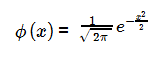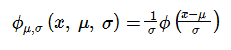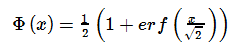## 12 September 2012

### Part 1: T-SQL Implementation of NORMDIST / NORM.S.DIST

This is Part 1 of a set of posts. The other posts are:
Part 1: Introduction
Part 2: Probability Density Function Implementation
Part 3: Cumulative Distribution Function Implementation 1
Part 4: Cumulative Distribution Function Implementation 2
Part 5: Cumulative Distribution Function Implementation 3
Part 6: References and Notes

# Introduction and Methods

I’m not the first to use Excel as a tool for developing an algorithm; nor the first, when implementing said algorithm, to be surprised that SQL Server does not ship with a library of statistical functions.
In this series of posts I’ll develop a set of T-SQL functions which implement the very useful Normal Distribution’s ‘Probability Density Function’ (PDF) and ‘Cumulative Distribution Function’ (CDF.)

### The Very Basics

The Standard Normal Distribution is the special case of a Normal Distribution with mean zero and standard deviation one. The function for the Standard Normal Distribution for variable x is:The Normal Distribution PDF (for a variable x with mean µ and standard deviation s) can be expressed as a function of the Standard Normal Distribution PDF:The function for the Standard Normal Distribution CDF is:Like the Normal Distribution PDF the Normal Distribution CDF can be expressed as a function of the Standard Normal Distribution CDF:Another way to express it is by means of the special function erf (the error function):### The Implementation

The Standard Normal Distribution PDF (and by extension the Normal Distribution PDF) can be implemented as a user defined function directly from the definition. Such an implementation is presented in Part 2 of this series. The Standard Normal Distribution CDF has no closed form equivalent and can only be approximated:
Part 3 presents an implementation of a simple (and fast) polynomial approximation with a precision slightly better than three decimal points.
Part 4 presents a more complex polynomial approximation implementation with a precision of seven decimal points.
Finally Part 5 presents an implementation using a set of rational approximation. This more complex and slower) approximation yields a precision of fifteen decimal digits, which is also the precision of a double precision floating point value.
The approximations in Part 3 & Part 4 are courtesy of Abramowitz & Stegun (1964) while the implementation in Part 5 uses an approximation of the erf function from W. J. Cody (1969.)
See Part 6 for a list of references and notes.

### Ensuring Accuracy

One of the problems when implementing mathematical functions is how to ensure the implementation is correct and the values returned accurate.
In order to test the accuracy of my implementations I've used to compute the values of doubles from -10 to 10 with 0.01 intervals and compared them to the results of the Excel 2010 implementation of the same function. Statistical functions in Excel versions prior to Excel 2003 where criticized for their accuracy. Microsoft greatly increased the accuracy of Excel 2003 from previous versions and made more improvements in Excel 2010.
Since the trigger for the work in these series of posts was my desire to implement an algorithm prototyped in Excel, and given that the latest Excel version addresses accuracy concerns; Excel 2010 is a good candidate to test the implementations.
I've also included the results using Excel 2007 as the reference implementation. In order to perform the comparison I wrote a small console utility called CheckError. CheckError gets as parameter the range, interval and functions to test and then:
• Writes on the console the maximum difference between Excel and Sql Server implementations and at which input value it occurrs.
• Writes files each containing the double precision floating point binary representation of:
• The input values.
• The Excel results.
• The Sql Server results.
• The differences between the Excel and Sql Server results.
• Writes a tab delimited file containing decimal string representations of the value above.
The binary files can be used to test the precision of the solution using other reference implementations (say MatLab.) The tab delimited files should not be used for this purpose as there is a loss of precision involved in converting from the binary representation to the decimal string representation.
I used the tab delimited file to create the graphs presented along side the implementations since the loss of precision is not relevant in this case.
The source code for CheckError the T-SQL implementations, output files and Excel file with graphs can be found at codeplex and is released under the Microsoft Public License (Ms-PL.)

### Caveat Emptor

The license for the code discussed here contains the full “as is” disclaimer, however since statistical functions may be used in all sorts of critical applications consider the following warnings if you decide to use these implementations:
While the tests described above are good enough for my purposes they are far from a complete analysis of the implementations.
In particular:
1. I have not performed a review of available literature to discover whether the sources I’ve based my implementations on have been disputed or corrected.
2. My implementation (or Excel 2010 which I use as the reference implementation or both) may have bugs which my testing does not reveal and may result in an error greater than the one stated.

### Note Regarding References

The implementations and testing tools presented here are my own, but no original research was undertaken in their development.
Part 6 contains a full list of references and notes on where I found what information.